# Catch up on the latest AI articles# Super Accelerated Translation Of High Resolution Images: ASAP-Net

3 main points
✔️ Significant speedup in image translation task compared to existing methods
✔️ Reduce the high resolution to operations at low resolution
✔️ Despite the speedup, the quality of the generated images is comparable to existing methods

Written
(Submitted on 5 Dec 2020)
1Accepted to arXiv.
Subjects: Computer Vision and Pattern Recognition (cs.CV)## First of all

The task of transforming an image from one domain to another has been extensively studied in recent years. Current approaches use conditional GANs to learn a direct mapping from one domain to the other. While these approaches have made rapid progress in terms of the quality of the visually generated images, they have also significantly increased the size and computational complexity of the models. This enormous computational complexity is a very serious problem when trying to use them in real-world applications.

In this paper, we develop a new architecture to speed up the task of image style transformation, named ASAP-Net (A Spartially-Adaptive Pixcelwise Network). (Figure 1).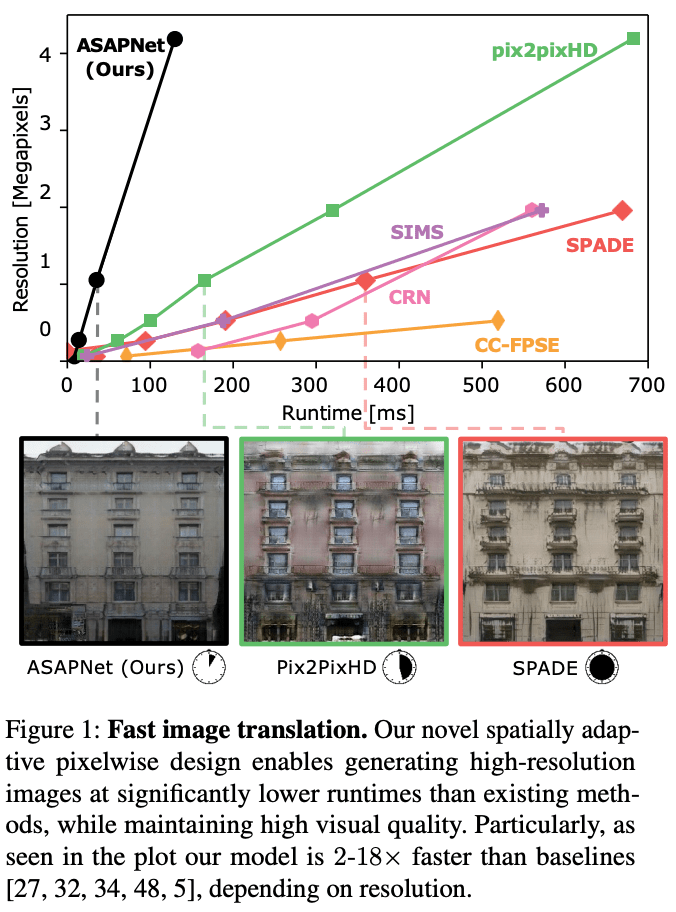## technique

As mentioned earlier, the task is to train the NN to perform a mapping between two image regions. That is, given an input image $x\in R^{H\times W\times 3}$, the generated image $y\in R^{H\times W\times 3}$ must look like it belongs to the target domain. The goal of this paper is to build a network that is more efficient than existing methods but still produces output with the same quality as existing methods.

Analyzing and understanding the contents of an image is a complex task and requires a correspondingly deep NN, but we believe in this paper that this analysis need not be done at full resolution. First, we synthesize high-resolution pixels using lightweight, parallelizable operators. We then perform the more costly image analysis at a very coarse resolution. This design minimizes the number of full-resolution computations and allows us to perform heavy incompressible computations with smaller inputs while still producing high-quality images.

The overall architecture of the proposed method is shown in Figure 3 below.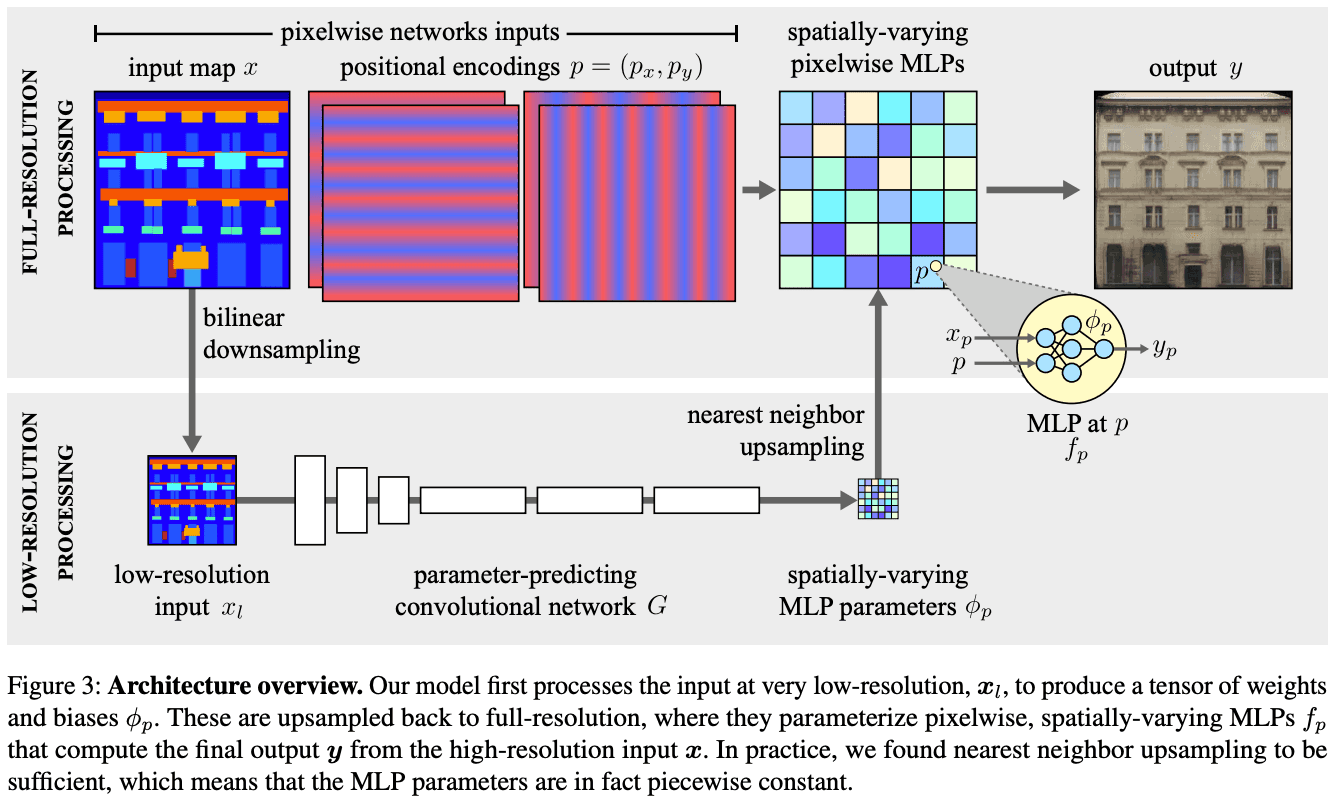### Pixelwise Networks

When the input is full resolution, the computational complexity is very high. To reduce the execution time, we model a pointwise nonlinear transformation $f_p$. Here, $p$ represents the coordinates of a pixel. This process corresponds to the top of Figure 3. Since each pixel is considered independently from other pixels, the computation can be parallelized. However, considering each pixel independently limits the expressive power of the model if it is not designed carefully.

We use two mechanisms to maintain spatial information:

1. The function $f_p$ for each pixel takes as input the color value $x_p$ plus the pixel coordinates $p$.
2. The per-pixel function $f_p$ is parameterized by the spatial variable $\phi_p$, specifically the multilayer perceptron (MLP), which defines the following mapping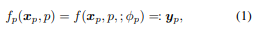In Equation (1), $f$ represents the MLP architecture (common to all pixels) and $\phi_p$ represents the MLP weights and biases (which vary spatially).MLPs have found that 5 layers and 64 channels per layer produce better quality images with shorter execution times.

If the input image is different, the set of parameters for each pixel is also different. Without the two properties of input adaptability and spatially varying parameters, the proposed method would be a poorly expressive model that is just a series of $1\times 1$ convolution layers.

### Predicting pixelwise network parameters from a low-resolution input

For adaptive transformation, $\phi_p$ must be a function of the input image. However, it is very difficult to predict the parameter vector $\phi_p$ for each pixel individually. Instead, it is predicted by a convolutional neural network $G$ on a much lower resolution image $x_l$. Specifically, the network $G$ outputs a grid of parameters with a resolution $S$ times smaller than $x$. This grid is upsampled using nearest-neighbor interpolation and becomes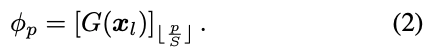As shown at the bottom of Figure 3, the low-resolution computation first downsamples $x$ bilinearly by a factor $S_1$ to obtain $x_l$. This image is then processed by $G$. $G$ and further reduced by the factor $S_2$. The final output of $G$ is a tensor with channels corresponding to the weights and biases of a per-pixel network with full resolution. Here we use $S_2=16$ and set $S_1$ so that $x_l$ has a maximum of 256 pixels. Thus, the total downsampling $S$ depends on the image size. This means that low-resolution processing can dramatically reduce the computational complexity of $G$ by processing very low-resolution representations of the input image.

The function parameter $\phi_p$ in the proposed method is predicted at low resolution and then upsampled. Therefore, the ability to predict the high resolution in detail is somewhat limited. In the proposed method, we avoid this by extending the per-pixel function $f_p$ to take the encoding of the pixel position $p$ as an additional input. spatially-varying MLPs such as CPPNs (Compositional Pattern-Producing Networks) can be trained more finely than parameter sampling. Moreover, we know that NNs have a spectral bias to learn low-frequency signals first. Therefore, instead of passing $p$ directly to the MLP as in Equation 1, we found it useful to encode each component of a 2D pixel position $p=(p_x,p_y)$ as a vector of sinusoids with a frequency higher than the upsampling factor. Each MLP has $2\times\times k$ additional inputs in addition to the pixel value $x_p$. This encoding corresponds to the upper part of Figure 3.

## Experiment

### Experiment setup

We verify the performance of the proposed method on an image translation task. In particular, we compare the execution time and image quality with previous studies.

• model
• Ours(ASAP-Net)
• CC-FPSE
• Introducing a new conditional convolutional layer to recognize semantic regions
• Transforming semantic images to realistic images using spatially adaptive normalization.
• pix2pixHD
• SIMS
• Improved segment structure
• CRN
• high-resolution conversion
• data set
• CMP
• 400 pairs of architectural images
• 360/40 sheets to divide into training/inference data
• 512*512,1024*1024
• Cityscapes
• Images of urban landscapes and their semantic label maps
• 3000/500
• 256*512,512*1024
• NYU depth dataset
• 1449 indoor landscape images
• 1200/249

### experimental results

inference time

The Nvidia GeForce 2080 ti GPU was used. Depending on the resolution, the proposed method is up to 6 times faster than pix2pixHD, and 18 times faster than SPADE, SIMS, and CRN. figure 1 shows that the superiority of the proposed method is seen at higher resolutions. Figure 1 shows that the superiority of the proposed method is observed at higher resolutions because the convolution stream of the proposed method is almost constant with respect to the image size at lower resolutions.Quality of the generated image

It can be seen that the generated images have a visual quality comparable to the baseline model.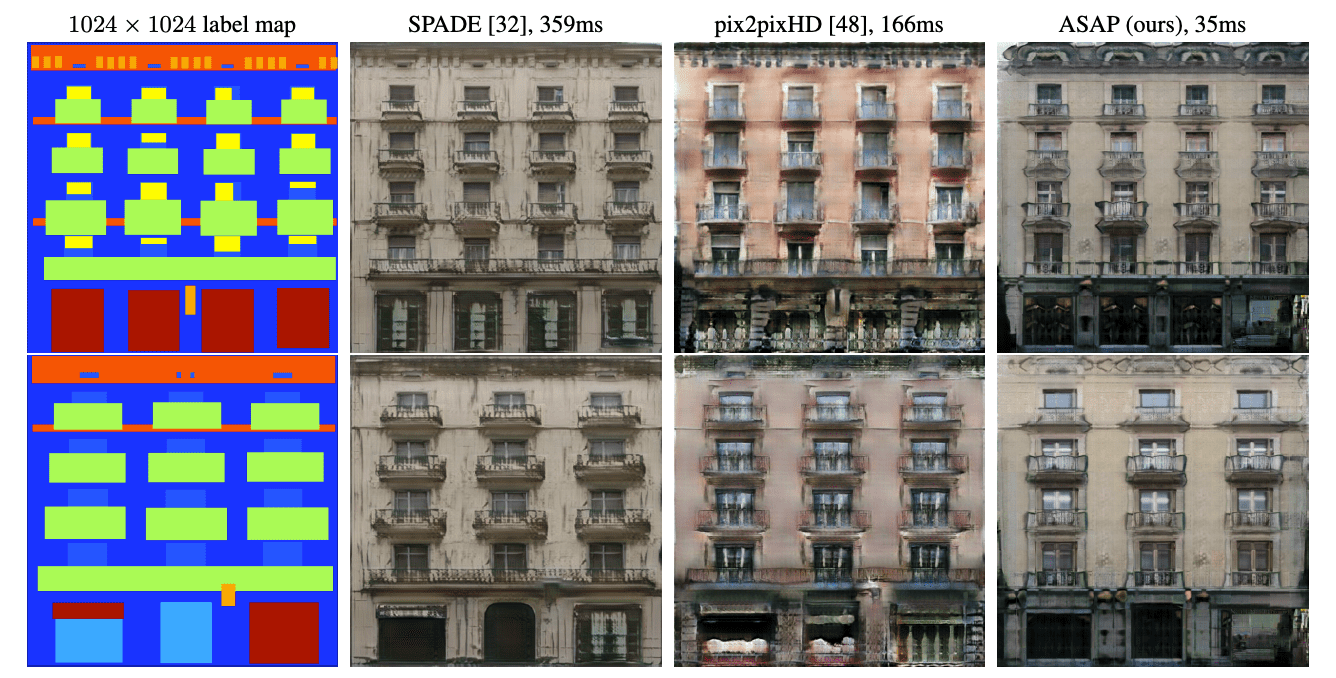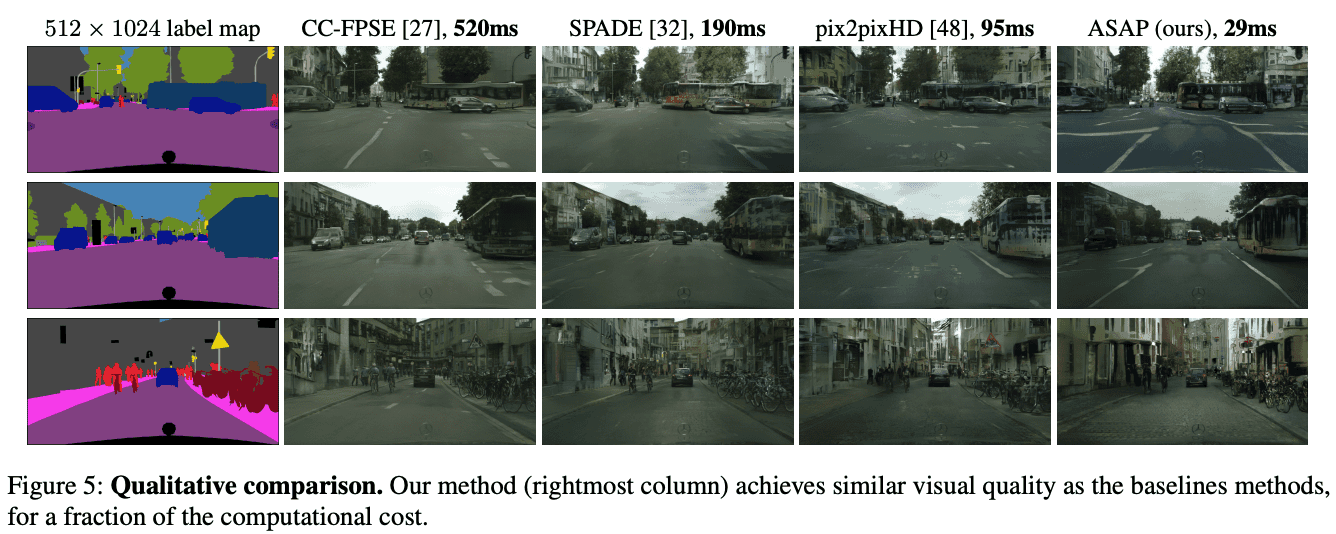Evaluation by humans

The AMT is used to evaluate the generated images by humans. We asked the users which of the generated images of the proposed method and the baseline model looks more realistic. Figure 7 shows that the users evaluate the proposed method as much as the baseline model.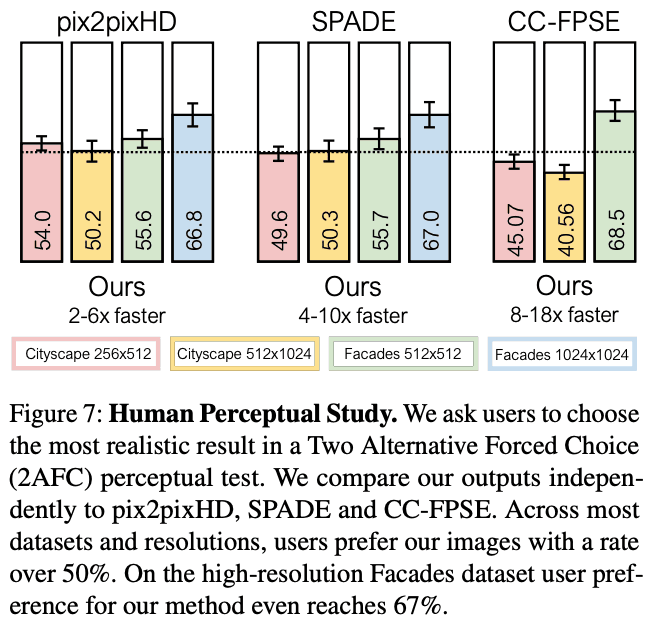Quantitative evaluation

We quantitatively evaluate the quality of the generated images. First, we pass the generated images through a semantic segmentation network and obtain FID scores and compare them. In Figure 8, we can see that the proposed method and pix2pixHD/SPADE have the same score. Figure 8 shows that the proposed method and pix2pixHD/SPADE have the same score, which means that the proposed method has the same quality as the baseline model, but the execution time is much shorter. When the number of parameters is reduced so that the baseline model has the same execution time as the proposed method, the FID score is significantly reduced.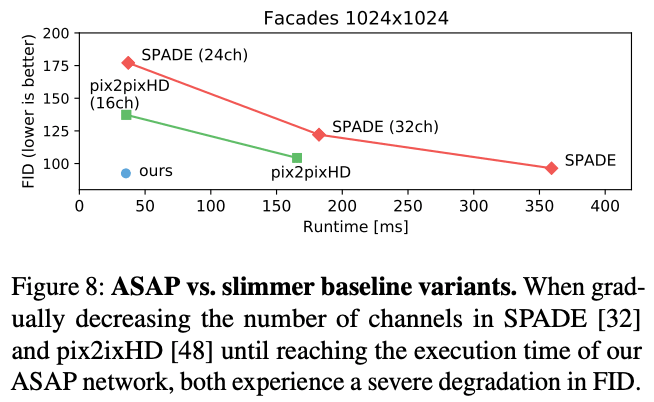## Summary

In this paper, we proposed an architecture that specifically focuses on speedup for the adversarial image transformation task. Despite the speedup by reducing the high-resolution image to a low-resolution process, we were able to produce images of comparable quality to existing methods. It is important to note that the computation is performed at low resolution and therefore the score can be slightly degraded by objects that are less than 3% of the image size.

If you have any suggestions for improvement of the content of the article,